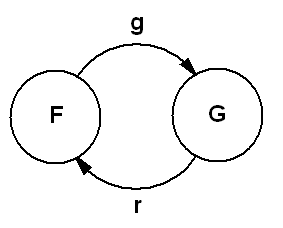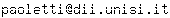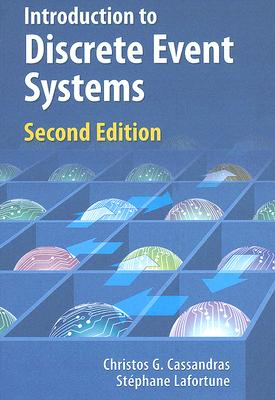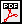Master of Science in Engineering University of Siena Automata and Queueing Systems Discrete Event Systems October 2015 - January 2016

## 1  News

• NEW! The results of the exam of July 25, 2016 are available in Section 4.3.
• See Section 4.1 for the learning assessment procedures.
• Students admitted to the oral exam are requested to send an email to the Instructor to schedule a date for the oral exam.
• The notes of the course are available in Section 5.1 (last update: October 27, 2015).

## 2  About the instructor

### 2.1  Instructor

 Simone Paoletti, PhD Assistant Professor Building: San Niccolò Floor: 2 Room: 229 (red circle on the map) Email:Phone: 0577 23 4850 ext. 1020 Web: http://www3.diism.unisi.it/~paoletti/

### 2.2  Office hours

• Thursday � from 2:30PM to 3:30PM (room 229)
• Friday � from 2:30PM to 3:30PM (room 229)

## 3  About the course

### 3.1  Training objectives

• Discrete event systems are systems whose dynamic behaviour is driven by asynchronous occurrences of discrete events.
• Examples can be found in a variety of fields, such as control, computer science, automated manufacturing, and communication and transportation networks.
• The aim of this course is to equip the students with several modelling, simulation and analysis tools for discrete event systems.
• Lab tutorials with Matlab will be an important part of this course.

### 3.2  Required background

• Dynamical systems
• Probability

### 3.3  Syllabus

 ALL ( ∼ 48 hours)
• Logical models of discrete event systems
• Timed models of discrete event systems
• Stochastic timed models of discrete event systems
• Continuous-time Markov chains
• Queueing theory
• Markovian queueing networks
 CAE-R&A only ( ∼ 24 hours)
• Discrete-time Markov chains
• Control applications of discrete event systems

### 3.4  Didactic methods

• The course will be organized in lectures (50%) and tutorials, held both in class (25%) and in computer lab (25%).

### 3.5  Reference book[CL08] C.G. Cassandras, S. Lafortune, "Introduction to discrete event systems". 2nd ed., Springer, 2008.

## 4  Exams

### 4.1  Learning assessment procedures

• The final exam consists of a written part (with the help of Matlab) and an oral part.
- Admission to the oral exam is subject to passing the written exam with a grade ≥ 18 (out of 30).
- The oral exam should be given within the same session as the written exam.
- In case of failure of the oral exam, the student must repeat also the written exam.
• The written part of the final exam can be substituted with:
- a midterm written exam (after first half of the course)
- a Matlab project (at the end of the course)
In this case, admission to the oral exam is subject to passing the midterm exam and the project with an average grade ≥ 18 and both grades ≥ 15 (out of 30).
• During the winter session of exams, it is allowed to enhance the grade of the midterm written exam.

### 4.2  Texts

 Past courses

## 6  About the lectures

### 6.1  Timetable

• Monday - from 11AM to 1PM (room F)
• Tuesday - from 9AM to 11AM (room F)
• Wednesday - from 10AM to 1PM (room 101)

### 6.2  Lecture schedule

 Date Topics Where to study (B = book; LN = lecture notes) Additional material October 5, 2015 (2h) Presentation of the course October 6, 2015 (2h) Basics of system theory Concept of system Static vs dynamical systems Concept of state Continuous vs discrete state Concept of event Time-driven vs event-driven systems Systems vs (mathematical) models Definition of Discrete Event System (DES) [B] Chapter 1 (except §1.2.7, §1.2.8 and §1.3.5)       [LN] Chapter 1 (except Examples 1.4 and 1.5) October 7, 2015 (3h) Examples of DES: queueing system cart system Untimed models of DES: state automata (with outputs)    Graphical representation of state automata Exercises [B] Section 2.2.2    [B] Examples 2.10 and 2.11    [LN] Examples 1.4 and 1.5    [LN] Chapter 2 Exercises (with solutions)October 12, 2015 (2h) Introduction to event timing Example: FIFO vs Round-Robin queueing [B] Section 5.1    [LN] Section 3.1 October 13, 2015 (2h) Definition of clock structure Timed models of DES: timed automata Examples of event timing dynamics [B] Section 5.2 (only §5.2.1) October 14, 2015 (3h) Algorithm for event timing dynamics Example: FIFO vs Round-Robin queueing (revisited) [B] Section 5.2 (only §5.2.2, §5.2.4 and §5.2.5) October 19, 2015 (2h) Exercises Exercises (with solutions)October 20, 2015 (2h) Review of probability theory [B] Appendix I (except §I.7 and §I.8) Test on probabilityOctober 21, 2015 (3h) Uncertainty sources in models of DES Models of DES with uncertainty: stochastic timed automata      Analysis of stochastic timed automata Example [B] Sections 6.1, 6.3 and 6.4 October 26, 2015 (2h) The exponential distribution: definition and properties October 27, 2015 (2h) Stochastic timed automata with Poisson clock structure Distribution of events Distribution of states Distribution of interevent times Distribution of state holding times [B] Section 6.8 October 28, 2015 (3h) The Poisson counting process Exercises [B] Sections 6.6 and 6.7 Exercises (with solutions)November 2, 2015 (2h) Exercises (continuation) November 3, 2015 (2h) Exercises Exercises (with solutions)November 4, 2015 (3h) Exercises Exercises (with solutions)November 9, 2015 (2h) Use of simulation for analysis of stochastic timed automata      Law of large numbers Estimation of state and event probabilities November 10, 2015 (2h) Use of simulation for analysis of stochastic timed automata      Histograms Estimation of probability density functions November 11, 2015 (3h) Exercises Exercises (with solutions)November 16, 2015 (2h) Midterm written exam November 17, 2015 (2h) Basics of stochastic processes Markov property and Markov processes Continuous-time homogeneous Markov chains Chapman-Kolmogorov equations [B] Section 6.2    [B] Sections 7.1 and 7.3 (only §7.3.1 and §7.3.4) November 18, 2015 (3h) Continuous-time homogeneous Markov chains Transition rate matrix and its properties State holding times Transition probabilities Estimation of transition rates [B] Section 7.3 (only §7.3.5, §7.3.6 and §7.3.7) November 23, 2015 (2h) Continuous-time homogeneous Markov chains State probabilities Graphical representation Classification of states [B] Section 7.3 (only §7.3.8 and §7.3.9) November 24, 2015 (2h) Continuous-time homogeneous Markov chains Steady state analysis Equivalences between classes of models Stochastic timed automata with Poisson clock structure    Continuous-time homogeneous Markov chains [B] Section 7.3 (only §7.3.10) November 25, 2015 (3h) Queueing theory Specification of queueing models A/B/m/K notation Transient and steady state analysis Characterization of steady state Performance of queueing systems Little's law PASTA property Examples of Markovian queueing systems [B] Sections from 8.1 to 8.6 (except §8.2.5) November 30, 2015 (2h) Introduction to Matlab (lab tutorial) December 1, 2015 (2h) Simulation of stochastic timed automata (lab tutorial) December 2, 2015 (2h) Exercises Exercises (with solutions)December 9, 2015 (3h) Discrete-time homogeneous Markov chains Chapman-Kolmogorov equations Transition probability matrix and its properties Example: a simplified telephone call process State holding times [B] Sections 7.1 and 7.2 (from §7.2.1 to §7.2.5) December 14, 2015 (2h) Discrete-time homogeneous Markov chains State probabilities Graphical representation Classification of states [B] Section 7.2 (from §7.2.6 to §7.2.8) December 15, 2015 (2h) Discrete-time homogeneous Markov chains Steady state analysis [B] Section 7.2 (from §7.2.9 to §7.2.10) December 16, 2015 (3h) Exercises Exercises (with solutions)December 21, 2015 (2h) Application: control of a machine subject to failures

File translated from TEX by TTH, version 4.03.
On 15 Nov 2016, 17:31.